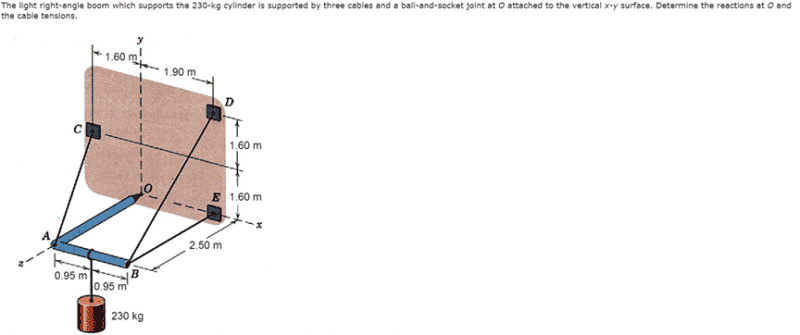# Statics problem involving moments and reaction forces

## Homework StatementThe right angle boom which supports the 230-kg cylinder is supported by three cables and a ball-and-socket joint at O attached to the vertical x-y surface. Determine the reactions at O and the cable tensions.

## Homework Equations

M [/B]= r x F
unit vector = (vector)/(magnitude of vector)
weight = weight of hanging mass = 230*9.81 = 2256.3 N

## The Attempt at a Solution

So far, I've gotten two of the reaction forces and two of the cable tensions. Using unit vectors, I was able to solve for the tension vectors:
T_ac = <-.4745, .4745, -.7414>T_ac
T_bd = <0, .7880, -.6156>T_bd
T_be = <0, 0, -1>T_be

Because the moment about an axis sums to zero:
ΣM_x = 0
0 = (weight of mass - .4745*T_ac - .7880*T_bd)*radius from axis
T_ac = (1/.4745)(2256.3 - .7880*T_bd)

ΣM_z = 0
0 = (T_bd*.7880 - .5*weight)*radius from axis
T_bd = 1431.66 N which goes to 1430 N (The homework site I'm using only allows three significant digits.)

T_ac = (1/.4745)(2256.3 - .7880*T_bd)
T_ac = 2377.56 N which goes to 2380 N

Up until this point, all the forces were equidistant from the axis in question.

Somewhere in here my numbers get messed up - the previous two tension forces are correct, but this one is wrong.

ΣM_y = 0
0 = T_ac*-.4745*2.5 - T_be*-1*1.9 - T_be*1.9 + .6156*1.9*T_bd
T_be = (1/1.9)(T_ac*.4745*2.5 - .6156*1.9*T_bd)
T_be = 606 N

Solving for the reaction forces:
ΣF_x = 0
0 = T_ac*-.4745 + O_x
O_x = 1130 N

ΣF_y = 0
0 = O_y + .4745*T_ac + T_bd*.7880 - weight
O_y = 0 N

These two reaction forces are correct, so if I'm doing something wrong up to this point, I'm making compensating errors. The next reaction force is incorrect, but it depends on T_be and since I know that number is wrong, even if my method here is correct, I won't be able to get the right answer.

ΣF_z = 0
0 = -.7414*T_ac - .6156*T_bd - T_be + O_z
O_z = 3250 N

I'm not sure what I'm doing wrong, but at this point I'd be happy to find any mistake!

SteamKing
Staff Emeritus
Homework Helper
Just giving you work a quick eyeball, TBD should have three non-zero components.

Also, when determining the sign of the components of a vector TBD, for example, these are usually calculated (xD - xB, yD - yB, zD - zB). [note the order]

I'm not quite sure I follow you. I calculated the unit vector for T_bd as follows:

B: <1.9, 0, 2.5>
D: <1.9, 3.2, 0>

BD = D - B
BD
= <0, 3.2, -2.5>
n_bd = <0, 3.2, -2.5> / (3.2^2 + 2.5^2)^.5
T_bd = n_bd * T_bd
T_bd = <0, .7880, -.6156>

Am I messing up the initial values for B and D?

SteamKing
Staff Emeritus
Homework Helper
I'm not quite sure I follow you. I calculated the unit vector for T_bd as follows:

B: <1.9, 0, 2.5>
D: <1.9, 3.2, 0>

BD = D - B
BD
= <0, 3.2, -2.5>
n_bd = <0, 3.2, -2.5> / (3.2^2 + 2.5^2)^.5
T_bd = n_bd * T_bd
T_bd = <0, .7880, -.6156>

Am I messing up the initial values for B and D?
No, it's my mistake here. I read the distance wrong from the diagram of the frame in the OP.SteamKing
Staff Emeritus
Homework Helper

## Homework Statement

View attachment 80357
The right angle boom which supports the 230-kg cylinder is supported by three cables and a ball-and-socket joint at O attached to the vertical x-y surface. Determine the reactions at O and the cable tensions.

## Homework Equations

M [/B]= r x F
unit vector = (vector)/(magnitude of vector)
weight = weight of hanging mass = 230*9.81 = 2256.3 N

## The Attempt at a Solution

So far, I've gotten two of the reaction forces and two of the cable tensions. Using unit vectors, I was able to solve for the tension vectors:
T_ac = <-.4745, .4745, -.7414>T_ac
T_bd = <0, .7880, -.6156>T_bd
T_be = <0, 0, -1>T_be

Because the moment about an axis sums to zero:
ΣM_x = 0
0 = (weight of mass - .4745*T_ac - .7880*T_bd)*radius from axis
T_ac = (1/.4745)(2256.3 - .7880*T_bd)

ΣM_z = 0
0 = (T_bd*.7880 - .5*weight)*radius from axis
T_bd = 1431.66 N which goes to 1430 N (The homework site I'm using only allows three significant digits.)

T_ac = (1/.4745)(2256.3 - .7880*T_bd)
T_ac = 2377.56 N which goes to 2380 N

Up until this point, all the forces were equidistant from the axis in question.

Somewhere in here my numbers get messed up - the previous two tension forces are correct, but this one is wrong.

My calculations for T_ac and T_bd agree with yours.ΣM_y = 0
0 = T_ac*-.4745*2.5 - T_be*-1*1.9 - T_be*1.9 + .6156*1.9*T_bd
T_be = (1/1.9)(T_ac*.4745*2.5 - .6156*1.9*T_bd)
T_be = 606 N

Why are you convinced that T_be is wrong?

Solving for the reaction forces:
ΣF_x = 0
0 = T_ac*-.4745 + O_x
O_x = 1130 N

ΣF_y = 0
0 = O_y + .4745*T_ac + T_bd*.7880 - weight
O_y = 0 N

These two reaction forces are correct, so if I'm doing something wrong up to this point, I'm making compensating errors. The next reaction force is incorrect, but it depends on T_be and since I know that number is wrong, even if my method here is correct, I won't be able to get the right answer.

ΣF_z = 0
0 = -.7414*T_ac - .6156*T_bd - T_be + O_z
O_z = 3250 N

I'm not sure what I'm doing wrong, but at this point I'd be happy to find any mistake!

I agree with your calculation of T_be. What is O_z supposed to be if 3250 N is incorrect?